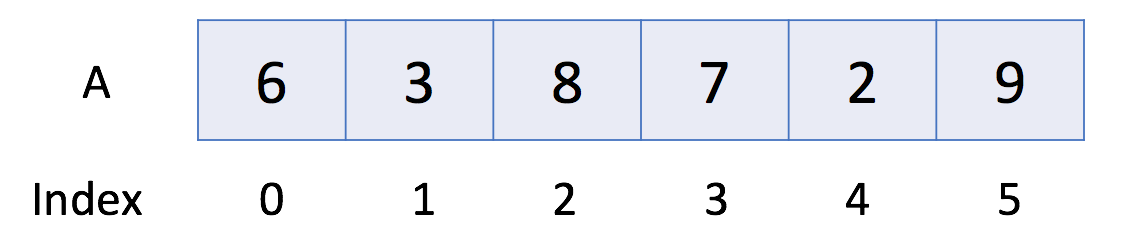• 关于网站更多信息请加QQ群(1061691290)
• jpress升级到4.x，显示有些问题，修复中
• 网站还会持续更新

## leetcode探索之数组和字符串学习 数组简介

1788人浏览 / 0人评论 / | 作者：因情语写  | 分类： 设计模式与算法  | 标签： 设计模式与算法  /  leetcode  |

本章节中，我们将介绍两个重要的概念：数组和动态数组。

这是你应当熟悉的基本数据结构。 我们也为你提供了使用内置的数组和动态数组的教程。

完成本章后，你将能够回答以下问题：

1. 数组和动态数组之间有什么不同？
2. 在你常用的语言中，数组和动态数组对应的内置数据结构是什么？
3. 如何在数组中执行初始化、数据访问、修改、迭代、排序等基本操作？
4. 如何在动态数组中执行初始化、数据访问、修改、迭代、排序、添加、删除等基本操作？

## 数组简介

数组是一种基本的数据结构，用于按顺序存储元素的集合。但是元素可以随机存取，因为数组中的每个元素都可以通过数组索引来识别。

数组可以有一个或多个维度。这里我们从一维数组开始，它也被称为线性数组。这里有一个例子：在上面的例子中，数组 A 中有 6 个元素。也就是说，A 的长度是 6 。我们可以使用 A 来表示数组中的第一个元素。因此，A = 6 。类似地，A = 3，A = 8，依此类推。

数组中的操作

``````// "static void main" must be defined in a public class.
public class Main {
public static void main(String[] args) {
// 1. Initialize
int[] a0 = new int;
int[] a1 = {1, 2, 3};
// 2. Get Length
System.out.println("The size of a1 is: " + a1.length);
// 3. Access Element
System.out.println("The first element is: " + a1);
// 4. Iterate all Elements
System.out.print("[Version 1] The contents of a1 are:");
for (int i = 0; i < a1.length; ++i) {
System.out.print(" " + a1[i]);
}
System.out.println();
System.out.print("[Version 2] The contents of a1 are:");
for (int item: a1) {
System.out.print(" " + item);
}
System.out.println();
// 5. Modify Element
a1 = 4;
// 6. Sort
Arrays.sort(a1);
}
}``````

## 动态数组简介

正如我们在上一篇文章中提到的，数组具有固定的容量，我们需要在初始化时指定数组的大小。有时它会非常不方便并可能造成浪费。

因此，大多数编程语言都提供内置的动态数组，它仍然是一个随机存取的列表数据结构，但大小是可变的。例如，在 C++ 中的 vector，以及在 Java 中的 ArrayList。

动态数组中的操作

``````// "static void main" must be defined in a public class.
public class Main {
public static void main(String[] args) {
// 1. initialize
List<Integer> v0 = new ArrayList<>();
List<Integer> v1;                           // v1 == null
// 2. cast an array to a vector
Integer[] a = {0, 1, 2, 3, 4};
v1 = new ArrayList<>(Arrays.asList(a));
// 3. make a copy
List<Integer> v2 = v1;                      // another reference to v1
List<Integer> v3 = new ArrayList<>(v1);     // make an actual copy of v1
// 3. get length
System.out.println("The size of v1 is: " + v1.size());;
// 4. access element
System.out.println("The first element in v1 is: " + v1.get(0));
// 5. iterate the vector
System.out.print("[Version 1] The contents of v1 are:");
for (int i = 0; i < v1.size(); ++i) {
System.out.print(" " + v1.get(i));
}
System.out.println();
System.out.print("[Version 2] The contents of v1 are:");
for (int item : v1) {
System.out.print(" " + item);
}
System.out.println();
// 6. modify element
v2.set(0, 5);       // modify v2 will actually modify v1
System.out.println("The first element in v1 is: " + v1.get(0));
v3.set(0, -1);
System.out.println("The first element in v1 is: " + v1.get(0));
// 7. sort
Collections.sort(v1);
// 8. add new element at the end of the vector
// 9. delete the last element
v1.remove(v1.size() - 1);
}
}``````

## 算法说明

### 寻找数组的中心索引

``````输入:
nums = [1, 7, 3, 6, 5, 6]

``````输入:
nums = [1, 2, 3]

• nums 的长度范围为 [0, 10000]。
• 任何一个 nums[i] 将会是一个范围在 [-1000, 1000]的整数。

``````// 想法：滑动窗口法，索引不断向后，前面加，后面减
public int pivotIndex(int[] nums) {
if(nums == null || nums.length < 3){
return -1;
}

int pre = 0;
int post = 0;

for(int i = 1; i < nums.length; i++){
post += nums[i];
}

if(pre == post){
return 0;
}

for(int i = 1; i < nums.length; i++){
pre += nums[i - 1];
post -= nums[i];

if(pre == post){
return i;
}
}

return -1;
}``````

算法已经很好了，是一种高效的算法。

### 至少是其他数字两倍的最大数

``````输入: nums = [3, 6, 1, 0]

6大于数组中其他元素的两倍。6的索引是1, 所以我们返回1.``````

``````输入: nums = [1, 2, 3, 4]

1. nums 的长度范围在[1, 50].
2. 每个 nums[i] 的整数范围在 [0, 99].

``````// 想法：找最大数，同时设置一个标志，看该最大数是否是上一个最大数的二倍或以上，同时，如果当前不满足2倍，则不要再处理非当前最大数，
// 如果满足2倍，则还要处理当前的非最大数
public int dominantIndex(int[] nums) {
if(nums == null){
return -1;
}

int max = nums;
boolean db = true;
int index = 0;

for(int i = 1; i < nums.length; i++){
if(max < nums[i]){
db = max << 1 <= nums[i];
index = i;
max = nums[i];
}else if(db){
db = nums[i] << 1 <= max;
}
}

return db ? index: -1;
}``````

db标志表示是否找到了题目要求的比其它数大两倍的数

再看一种比较直观的算法

``````public int dominantIndex(int[] nums) {
int res = 0;
int max = 0;
for (int i = 0; i < nums.length; i++) {
if (nums[i] > max) {
res = i;
max = nums[i];
}
}
for (int i = 0; i < nums.length; i++) {
if (max < 2 * nums[i] && max != nums[i]) {
return -1;
}
}

return res;
}``````

上面先找出最大数，再计算最大数是否不小于其它数的两倍，不满足返回-1，满足返回找到的数的索引

### 加一

``````输入: [1,2,3]

``````输入: [4,3,2,1]

``````// 想法：最低位加1后，模10的结果作为当前位的数，除10的结果（0或1）作为进位，其他位加前一位的进位（0或1），这位也
// 和初始的操作统一起来，初始看成进位是1
public int[] plusOne(int[] digits) {
int extendLength = 1;
for(int i = 0; i < digits.length; i++){
if(digits[i] != 9){
extendLength = 0;
break;
}
}

int[] newDigits = new int[digits.length + extendLength];
int temp = 1;
for(int i = digits.length - 1; i >= 0; i--){
temp = digits[i] + temp;
newDigits[i + extendLength] = temp%10;
temp = temp/10;
}

if(extendLength == 1){
newDigits = 1;
}

return newDigits;
}``````

代码先判断了会不会使最终结果进一位（如9999），还有优化的空间，当某一位时的进位是0时，就可以停止循环并返回了

再看一种方法

``````public int[] plusOne(int[] digits) {
int  k=digits.length;
for (int i=k-1;i>=0;i--)
{
digits[i]++;
digits[i]=digits[i]%10;
if (digits[i]!=0)
return digits;
}
digits= new int[k+1];
digits=1;
return digits;

}``````

上面的方法好处是不用每一位都计算，如果某一位没有进位就停止了

#### 作者其它文章